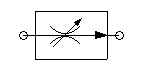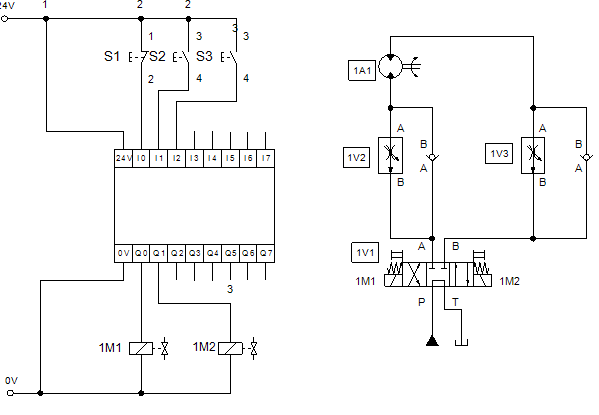# (English) Flow control valve

(English)

### Flow control valveSymbol - Flow control valve

The flow control valve is used for load-independent control of the volume flow. That means that the speed of a cylinder or the rotational speed of a hydraulic motor can be kept constant even under different load. Let´s explain the function:Hydraulics - Flow Control Valve - how it works

In order to keep the volume flow constant, the hydraulic pressure drop at the throttle Δp = p2 -p1 must be constant. The condition Δp = constant is fulfilled when the volume flow also is constant. With a changing load on the actuator, the control piston shifts and thereby reduces or increases the opening degree for the volume flow.

Example: If the load on the motor increases the pressure p2 increases immediately. Via the control line the pressure on the area A2 rises accordingly. As a result the control piston opens and thus counteracts a decrease in the volume flow.

As the flow rate increases, so does the pressure p1 and the pressure drop Δp remains constant because the control piston has stabilized to the new load.

Review the control position in balance:

F1 = F2

A1 * p1 = A2 * p2 + Fspring

Because A = A1 = A2 we can simplify:

A * p1 = A * p2 + Fspring

A * p1 - A * p2 = Fspring

A (p1 - p2) = Fspring

p1 –p2 = Δp = Fspring/A

=>  Δp is independent of p1 or p2!

Work order:  Hydraulic motor speed control with a flow control valveHydraulic flow control valve application

(English)